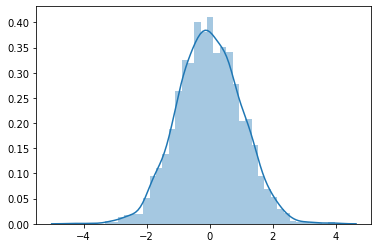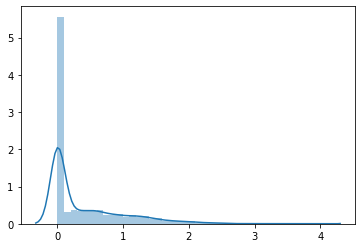# Doubtful ReLU use

when training a neural network, if we pass our input through ReLU, then it makes negative values zero, but this does not lead to a normal or symmetric distribution, for example,

``````import torch, seaborn
input = torch.randn(1, 3, 28, 28)
rlu = nn.ReLU()
seaborn.distplot(input.view(-1).detach().numpy())
``````

givesafter applying ReLU,

``````seaborn.distplot(rlu(input).view(-1).detach().numpy())
``````

this distribution becomes,do we not want to preserve symmetry of this distribution during training?

same thing happens when we use,

``````g = nn.CELU()
seaborn.distplot(g(((input))).view(-1))
````````````h = nn.ELU()
seaborn.distplot(h(((input))).view(-1))
````````````i = nn.SELU()
seaborn.distplot(i(((input))).view(-1))
``````Hi Ravi!

Well, the short answer is that `ReLU` (and similar activations) work
well for various neural-network models, so people keep using them.

But there is some intuition and mathematical reasons behind some
of this.

Let me start from the opposite direction: Why would you want normal
or symmetric distributions?

While one can imagine problems where the inputs to the neural network
are approximately normally distributed, there are many real-world
problems where this is not the case. And even for problems where the
inputs are normally distributed, why would you want the final outputs
or outputs of intermediate layers to be normal?

Now for some concrete reasons:

Non-linear activations are essential to how neural networks work.
(Two linear layers in a row without a non-linear activation in between is
just a single linear layer with some potentially redundant parameters.)

I’m sure some mathematician will prove me wrong, but if you apply a
non-linear function to a normal (or gaussian) distribution, you cannot
get a normal (or gaussian) distribution back. Neural networks won’t
work without non-linear activations, so they have to have non-normal
distributions.

There are, of course, symmetric non-linear activations – `tanh()`
is symmetric, and `sigmoid()` is symmetric with shift. But, again,
empirically non-symmetric activations often work better.

The intuition I’ve heard about `ReLU` in particular is that when the
training drives the (inputs to) `ReLU` into the negative range (that
gets mapped to zero), the `ReLU` “turns off” and your network
becomes more sparse (mathematically speaking, but not in terms
of storage or computation). And the sparseness supposedly can
help the network to “generalize” better.

Best.

K. Frank

1 Like

Yes, I think it is used to have sparse representations.
I think goal is to simulate how neurons in our brain work to accomplish image classification, that is we have bunch of neurons, but most of them stay inactive, and only a few of them fire at a time, that is why I see dropout also being used.